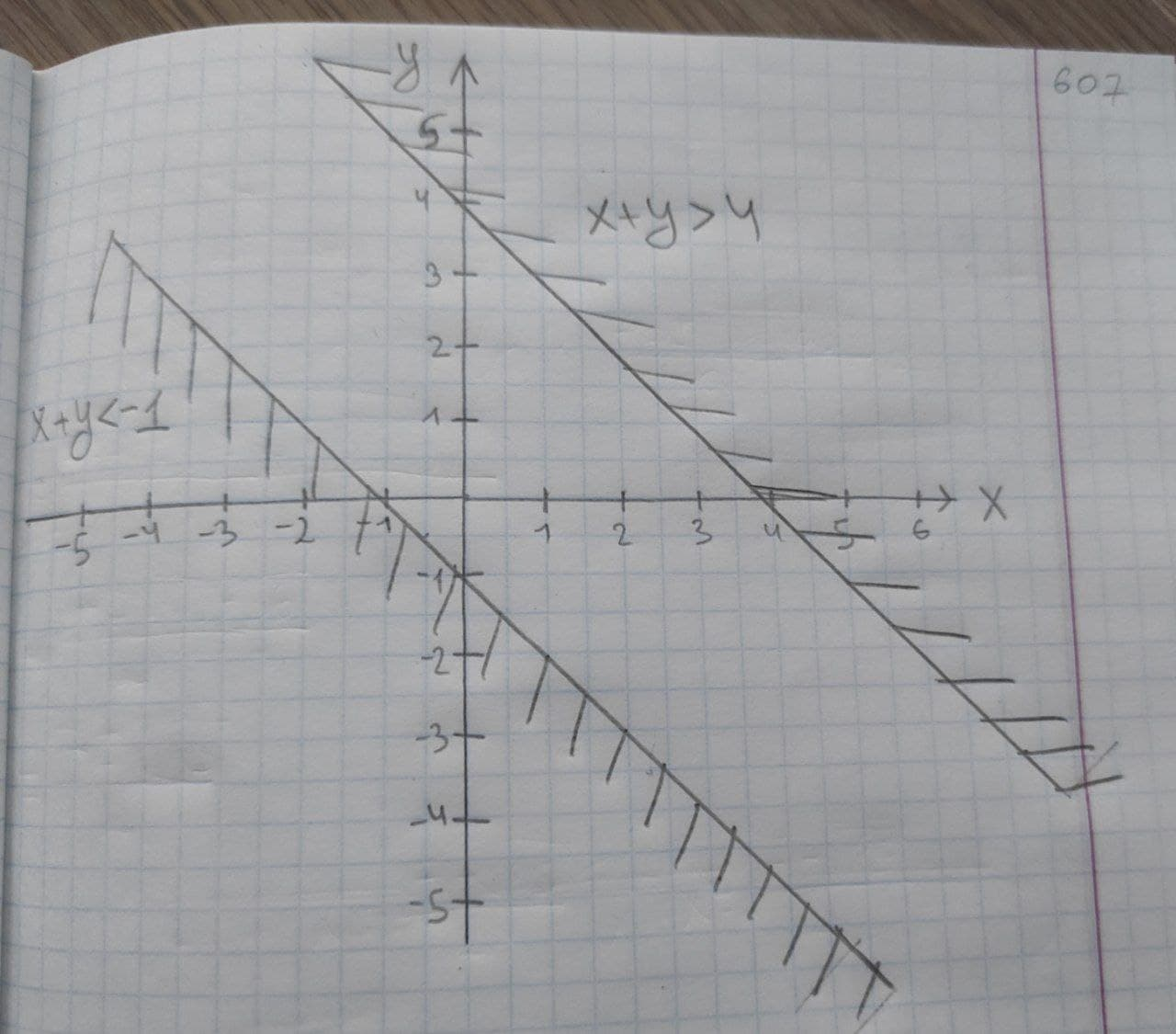# Graph the solution set of the system of inequalities or indicate that the system has no solution.{(x+y>4),(x+y<-1):}jernplate8 2020-10-21 Answered

Graph the solution set of the system of inequalities or indicate that the system has no solution.
$\left\{\begin{array}{c}x+y>4\\ x+y<-1\end{array}$

You can still ask an expert for help

## Want to know more about Inequalities systems and graphs?

• Questions are typically answered in as fast as 30 minutes

Solve your problem for the price of one coffee

• Math expert for every subject
• Pay only if we can solve itUsamah Prosser
System of inequalities will have solutions if they have common region when we graphed them.
From the given equations of inequalities we can find that they contradict to each other.
We will check this by drawing the graph of the given inequalities.
To graph the inequalities, first we will convert them into standard equation.
x+y=4 and x+y=−1
Here, both the equations are parallel to each other.From the graph, we can find that there is no region which is common to both.
It means that the given system of inequalities have no solutions.
Hence, given system of inequalities has no solutions.